2021-2022学年初中七年级第七单元数学练习题含解析
2021-2022学年初中七年级第七单元数学练习题含解析

2022-04-30

1.

A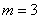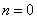B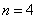C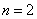D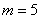【答案】

A

【解析】

【分析】

【详解】

【点睛】

2.

A 5 B 4 C 3 D 2

【答案】

D

【解析】

【分析】

【详解】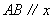轴，

a +3=4

A 3 4 ），点 B 1 4 ），

AB =3-1=2

【点睛】

3.

A 5 B 6 C 7 D 8

【答案】

D

【解析】

【分析】

【详解】

m -2=0 ，解得： m =2

P y 轴正半轴上，且到原点 O 的距离为 6

∴2 m + n =6

∴2×2+ n =6

n =2

m =2 n =2 时，

m +3 n =2+3×2=8

【点睛】

4.

A 2 B 3 C 4 D 5

【答案】

C

【解析】

【分析】

【详解】

k =2 时， x 2 - x 1 =1-5(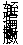-0)=1,

x 2 =1+1=2,

y 2 = y 1 +[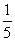]=1

P 2 2 1 ），

k =3 时， x 3 - x 2 =1-5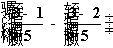=1

x 3 =1+2=3

y 3 = y 2 +[]=1

P 3 3 1 ），

k =4 时， x 4 - x 3 =1-5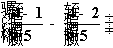=1

x 4 =3+1=4

y 4 = y 3 +[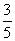]-[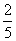]=1

P 4 4 1 ），

k =5 时， x 5 - x 4 =1-5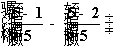=1

x 5 =4+1=5

y 5 = y 4 +[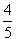]-[]=1

P 5 5 1 ），

k =6 时， x 6 - x 5 =1-5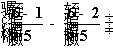=1

x 6 =5+1-5=1

y 5 = y 4 +[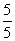]-[]=1+1=2

P 6 (1 2)

7≤ k ≤10 时， P 7 P 8 P 9 P 10 的坐标分别为（ 2,2 ），（ 3,2 ），（ 4,2 ），（ 5 3 ），

k =11 时， x 11 - x 10 =1-5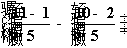=1

x 11 =1

y 11 =3

P 6 (1 3)

12≤ k ≤15 时， P 12 P 13 P 14 P 15 的坐标分别为（ 2,3 ），（ 3,3 ），（ 4,3 ），（ 5 3 ），

k =5 ,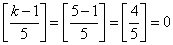，故甲正确；时，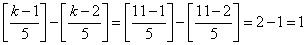，故乙正确；

6 棵树种植在点 P 6 (1 2) 处，故丙不正确；

1-5 棵，纵坐标均为 1,6-10 棵纵坐标均为 2 ，每行种植 5 棵树，故丁正确；

2022=404×5+2 ，第 2022 棵树的位置为 404 行第 2 ．故戊正确；

【点睛】

5.

A ．第一象限 B ．第二象限 C ．第三象限 D ．第四象限

【答案】

A

【解析】

【分析】

【详解】

【点睛】40

22
55.00%

5
12.5%

12
30.0%

1
2.5%

20
50.0%

20
50.0%

3
7.5%

28
70.0%

1
2.5%

3
7.5%

3
7.5%

1
2.5%

1
2.5%

0